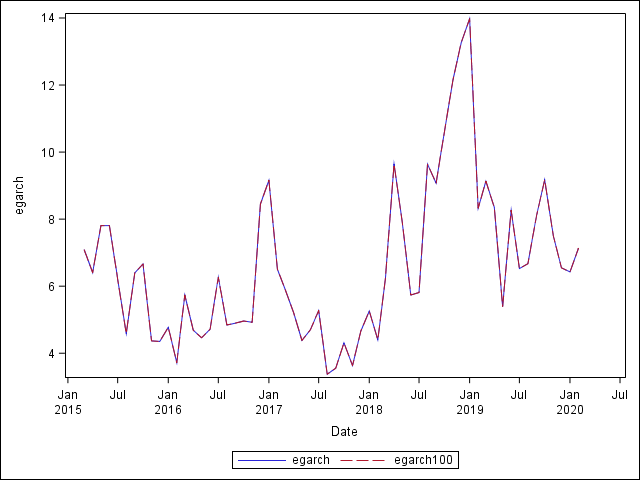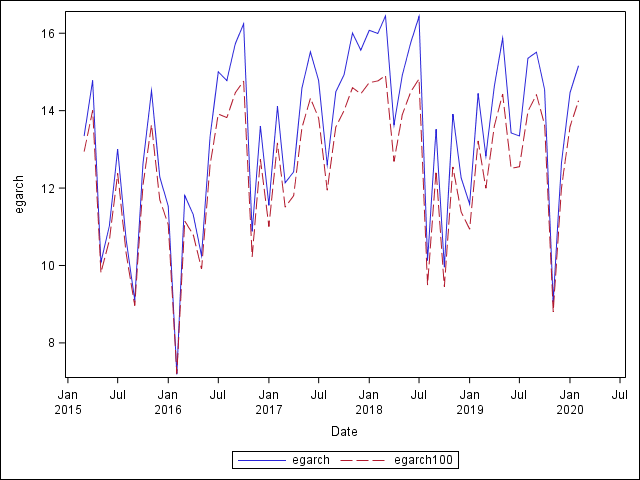## Is AUTOREG Sensitive to Scaling?

One can estimate GARCH using either raw numbers (0.1234) or percent ones (12.34). This code downloads most recent 60 monthly rate of returns of Facebook from Yahoo, estimates EGARCH using both raw and percent, and plots them together.

``````filename ret url 'https://query1.finance.yahoo.com/v7/finance/download/FB?period1=1337299200&period2=1584489600&interval=1mo&events=history';

proc import file=ret dbms=csv replace out=ret(where=("1jan2015"d<date<"1mar2020"d));
run;

proc expand out=ret;
id date;
run;

proc autoreg noprint;
model ret=/garch=(q=1,p=1,type=exp) maxiter=5000;
output ht=egarch out=egarch;
model ret100=/garch=(q=1,p=1,type=exp) maxiter=5000;
output ht=egarch100 out=egarch100;
run;

data egarch;
merge egarch egarch100;
by date;
egarch=sqrt(egarch);
egarch100=sqrt(egarch100)*100;
run;

ods listing close;
ods results=off;
ods listing gpath="!userprofile\desktop\";
ods graphics/reset;

proc sgplot;
series x=date y=egarch;
series x=date y=egarch100;
run;

ods results=on;
ods listing;``````

As expected, there is marginal difference between the raw and percent numbers.This is not always the case. Sometimes they differ from one another. The code allows to switch from Facebook to Twitter by turning on (off) the second (first) line. Though their difference is still endurable, I sometimes see dramatic numerical difference due to the raw and percent numbers.Which number is numerically better (stable?) to estimate those volatility models in SAS? I personally prefer percent numbers with no specific reason currently, so want to further clarify this point.

## Re: Is AUTOREG Sensitive to Scaling?

This question has been answered via Technical Support track and below is a summary of the answer to this question:

Yes, PROC AUTOREG can be sensitive to scaling when estimating GARCH models, and whether you use percentage numbers or raw numbers is more a personal choice. In some cases using percentage numbers may be more stable in estimation than using the raw numbers, possibly due to cutting off of the decimal part in the optimizer.

Discussion stats